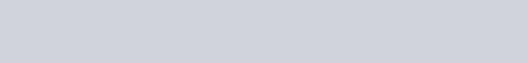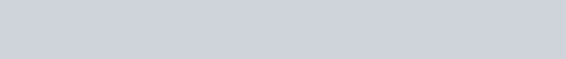The Modified Dietz rate of return attempts to estimate a money-weighted rate of return (MWRR) by weighting each cash flow by the proportion of the measurement period it is present or absent from the portfolio.

Similar to the money-weighted rate of return, the calculation requires the investor to know the portfolio values at the start and end of the measurement period, as well as the cash flow amounts and dates when each cash flow occurs.  Unlike the MWRR, the calculation does not require an exhaustive trial and error procedure, or sophisticated computing power.Source:  CFA Institute

The Modified Dietz rate of return can differ substantially from the time-weighted rate of return (TWRR) when large cash flows occur during periods of significantly fluctuating portfolio values (just like the money-weighted rate of return).  This makes the Modified Dietz rate of return less ideal for benchmarking portfolio managers or strategies than the TWRR.  For example:

• When a large contribution is made prior to a period of relatively good (bad) performance, the Modified Dietz rate of return (ModDietz) will overstate (understate) a portfolio’s performance, relative to the time-weighted rate of return (TWRR).
• When a large withdrawal is made prior to a period of relatively good (bad) performance, the Modified Dietz rate of return (ModDietz) will understate (overstate) a portfolio’s performance, relative to the time-weighted rate of return (TWRR).

Using the values from our original example, we would plug in the appropriate numbers and calculate the rate of return for each investor.

Example:  Calculation of wiExample:  Modified Dietz Rate of Return (MDRR) – Investor 1Example:  Modified Dietz Rate of Return (MDRR) – Investor 2Performance Results

 Methodology Investor 1 Investor 2 Time-Weighted Rate of Return (TWRR) 9.79% 9.79% Money-Weighted Rate of Return (MWRR) 8.98% 10.64% Modified Dietz Rate of Return (ModDietz) 8.97% 10.66%

As we can see in the chart above, the Modified Dietz rate of return is nearly identical to the money-weighted rate of return.  In my final blog post of the series, we will examine how calculating the Modified Dietz rate of return over monthly time periods can help an investor better estimate the time-weighted rate of return.